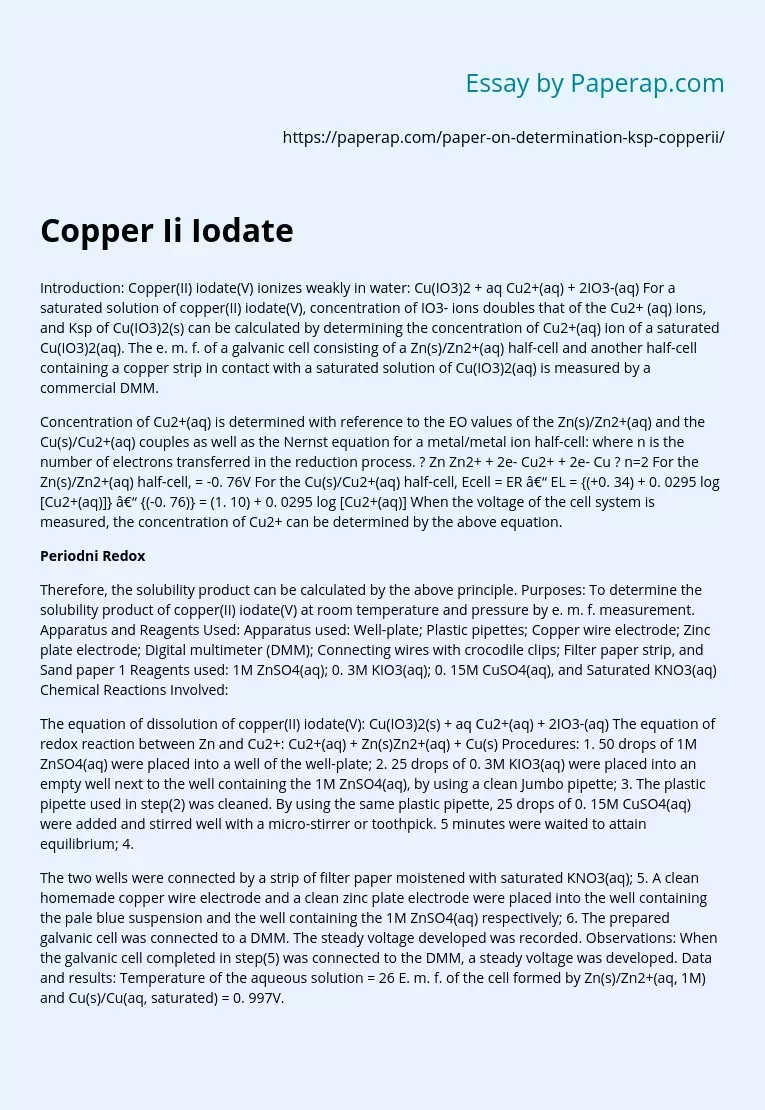# Copper Ii Iodate

Introduction: Copper(II) iodate(V) ionizes weakly in water: Cu(IO3)2 + aq Cu2+(aq) + 2IO3-(aq) For a saturated solution of copper(II) iodate(V), concentration of IO3- ions doubles that of the Cu2+ (aq) ions, and Ksp of Cu(IO3)2(s) can be calculated by determining the concentration of Cu2+(aq) ion of a saturated Cu(IO3)2(aq). The e. m. f. of a galvanic cell consisting of a Zn(s)/Zn2+(aq) half-cell and another half-cell containing a copper strip in contact with a saturated solution of Cu(IO3)2(aq) is measured by a commercial DMM.

Concentration of Cu2+(aq) is determined with reference to the EO values of the Zn(s)/Zn2+(aq) and the Cu(s)/Cu2+(aq) couples as well as the Nernst equation for a metal/metal ion half-cell: where n is the number of electrons transferred in the reduction process. ? Zn Zn2+ + 2e- Cu2+ + 2e- Cu ? n=2 For the Zn(s)/Zn2+(aq) half-cell, = -0.

76V For the Cu(s)/Cu2+(aq) half-cell, Ecell = ER – EL = {(+0. 34) + 0. 0295 log [Cu2+(aq)]} – {(-0. 76)} = (1. 10) + 0. 0295 log [Cu2+(aq)] When the voltage of the cell system is measured, the concentration of Cu2+ can be determined by the above equation.

## Periodni Redox

Therefore, the solubility product can be calculated by the above principle. Purposes: To determine the solubility product of copper(II) iodate(V) at room temperature and pressure by e. m. f. measurement. Apparatus and Reagents Used: Apparatus used: Well-plate; Plastic pipettes; Copper wire electrode; Zinc plate electrode; Digital multimeter (DMM); Connecting wires with crocodile clips; Filter paper strip, and Sand paper 1 Reagents used: 1M ZnSO4(aq); 0.

Get quality help nowKarrieWritesVerified

Proficient in: Chemistry5 (339)

“ KarrieWrites did such a phenomenal job on this assignment! He completed it prior to its deadline and was thorough and informative. ”+84 relevant experts are online

3M KIO3(aq); 0. 15M CuSO4(aq), and Saturated KNO3(aq) Chemical Reactions Involved:

The equation of dissolution of copper(II) iodate(V): Cu(IO3)2(s) + aq Cu2+(aq) + 2IO3-(aq) The equation of redox reaction between Zn and Cu2+: Cu2+(aq) + Zn(s)Zn2+(aq) + Cu(s) Procedures: 1. 50 drops of 1M ZnSO4(aq) were placed into a well of the well-plate; 2. 25 drops of 0. 3M KIO3(aq) were placed into an empty well next to the well containing the 1M ZnSO4(aq), by using a clean Jumbo pipette; 3. The plastic pipette used in step(2) was cleaned. By using the same plastic pipette, 25 drops of 0. 15M CuSO4(aq) were added and stirred well with a micro-stirrer or toothpick. 5 minutes were waited to attain equilibrium; 4.

The two wells were connected by a strip of filter paper moistened with saturated KNO3(aq); 5. A clean homemade copper wire electrode and a clean zinc plate electrode were placed into the well containing the pale blue suspension and the well containing the 1M ZnSO4(aq) respectively; 6. The prepared galvanic cell was connected to a DMM. The steady voltage developed was recorded. Observations: When the galvanic cell completed in step(5) was connected to the DMM, a steady voltage was developed. Data and results: Temperature of the aqueous solution = 26 E. m. f. of the cell formed by Zn(s)/Zn2+(aq, 1M) and Cu(s)/Cu(aq, saturated) = 0. 997V.

Discussions: 1. The expression of the Ksp of copper(II) iodate(V) = [Cu2+(aq)]eqm [IO3-]2eqm 2. By the equation evaluated in the introduction, Ecell = (1. 10) + 0. 0295 log [Cu2+(aq)] = 0. 997V 0. 0295 log[Cu2+(aq)] = -0. 103 log[Cu2+(aq)] = -3. 492 ? [Cu2+(aq)]eqm = 3. 225 i? 10-3 moldm-3 Concentration of Cu2+(aq) in the saturated pale blue suspension = 3. 225  10-3 moldm-3 3. By the equation of dissolution of copper(II) iodate(V), Cu(IO3)2(s) + aq Cu2+(aq) + 2IO3-(aq) [Cu2+(aq)] : [IO3-(aq)] = 1 : 2 ?[IO3-(aq)]eqm = 2 i? 3. 225 i? 10-3 = 6. 450  10-3 moldm-3 Concentration of IO3-(aq) in the saturated pale blue suspension = 6.

450  10-3 moldm-3 4. The value for Ksp of Cu(IO3)2 = [Cu2+(aq)]eqm [IO3-]2eqm = (3. 225 10-3)  (6. 450  10-3)2 = 1. 34  10-10 mol3dm-9 5. From the data of the Faculty of Chemistry and Technology, University of Split, the literature value of Ksp of Cu(IO3)2 is 6. 94 i? 10-8 mol3dm-9 . Obviously, the literature value was much larger than the experimental value. The discrepancy came from several reasons: 1 The experimental temperature was 26? , which was different from the expected room temperature 25?. As temperature is the main factor of Ksp, the discrepancy of Ksp was caused by the difference of temperature;

2 The low quality of copper and zinc electrode may affected the e. m. f. taken from the DMM, so the Ksp result was also affected; 3 The concentration of solutions prepared may be different with the expected value. The e. m. f. given out may not equal to the theoretical values, so the Ksp obtained may be different. Conclusion: By the e. m. f. method, the solubility product of copper(II) iodate(V) at room temperature and pressure was determined. Ksp for = 1. 34 i? 10-10 mol3dm-9 Reference:  : Solubility Product Constants, Faculty of Chemistry and Technology, University of Split http://www. ktf-split. hr/periodni/en/abc/kpt. html .